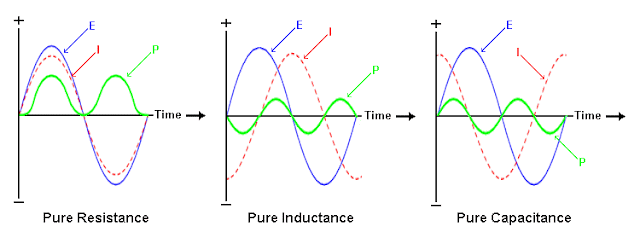Figure 1. Power Triangle

The Power Triangle in AC System

1. Real Power (P) - Real power is expressed in Watts (W).
• In electrical heating equipment this is the actual power that is being converted directly to heat. In terms of electrical machines, this is the power that is being converted directly to mechanical energy.
2. Reactive Power (Q)- Reactive power is expressed in VAR (Reactive Volt-Ampere).
• This power is mostly drawn by inductor and capacitor.
3. Apparent Power (S) - Apparent power is expressed in VA (Volt-Amperes).
• Apparent power is the power that is actually being drawn by the network operating in Alternating Current system.
• We can say that there might be two separate AC systems that draws exactly the same real power but draws different apparent power.
These three quantities can be represented by a power triangle as describe in figure 1. We can analyze the relationship of these quantities like the analysis in right triangle by which the trigonometric formula can be use. Using such an analysis we can say that

• S^2 = P^2+ Q ^2 <formula.1>
• cos Θ =  P / S or WATTS /VA  <formula.2>, also known as the power factor
• Θ = cos^ (1-)      <formula.3> , also known as the power factor angle

For some consideration power factor can be analyze like an efficiency of a system.Three types of power factor
• Lagging Power Factor- when the voltage lags behind the current as the two alternating waves propagate. This happens in inductive loads.
• Leading Power Factor - when the current leads the voltage as the two alternating waves propagates. This happens in capacitive loads.
• Unity Power Factor- when the voltage and current waves simultaneously arrives in x- coordinate. This happens in a purely resistive loads or when the capacitive load will equal to that of the inductive load in the system.Figure 2. Voltage and Current wave propagation in time-magnitude coordinate
Furthermore we can expressed these power in the following formula:
• P= 3 VpIp cos Θ or √3 VL IL cos Θ    <formula 4> for real power 3 phase
• Q= 3 VpIp sin Θ or √3 VL IL sin Θ    <formula 5> for reactive power, 3phase
• S = 3 VpIp or √3 VL IL                   <formula 6> for apparent power, 3 phase

Example:

Two separate 3 phase electrical system delivers the same amount of power of 10,000 watts. System #1 has a power factor of .60 while the other (system #2) has a power factor of 0.85. Determine the current drawn by each systems? (the 2 systems uses the same line voltages, 440 volts)

Solution:

System #1,

• P = √3 VL IL cos Θ ;  cos Θ = power factor = 0.6
• IL = 10,000 / (√3 * 440 * .6)
• IL= 21.87 Amperes

System #2,
• P = √3 VL IL cos Θ ;  cos Θ = power factor = 0.85
• IL = 10,000 / (√3 * 440 * .85)
• IL= 15.44 Amperes

In the above example the two networks delivered the same amount of useful power but the other network is more efficient compare to the others. This is because of power factor.

Therefore, we can say that two networks may deliver the same useful power (WATTS) but differs in the consumption of the apparent power --- one is high and the other is low.